# How to Calculate and Solve for Glass Gas Solubility Parameter in a Fluid | Nickzom Calculator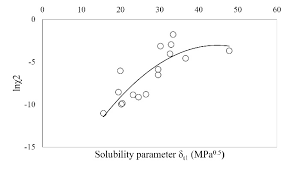The image above represents glass gas solubility parameter.

To compute for the glass gas solubility parameter, one essential parameter is needed and the parameter is Pressure (P).

The formula for calculating the glass gas solubility parameter:

x = 2.8869 – [14.1811 – 3.3093logP]0.5

Where:

x = Glass Gas Solubility Parameter, x
P = Pressure

Let’s solve an example;
Find the glass gas solubility parameter when the pressure is 62.

This implies that;

x = Glass Gas Solubility Parameter, x = 62

x = 2.8869 – [14.1811 – 3.3093logP]0.5
x = 2.8869 – [14.1811 – 3.3093log(62)]0.5
x = 2.8869 – [14.1811 – 3.3093(1.79)]0.5
x = 2.8869 – [14.1811 – 5.93]0.5
x = 2.8869 – [8.249]0.5
x = 2.8869 – 2.87
x = 0.0146

Therefore, the Glass Gas Solubility Parameter is 0.0146.

Nickzom Calculator – The Calculator Encyclopedia is capable of calculating the glass gas solubility parameter.

To get the answer and workings of the glass gas solubility parameter using the Nickzom Calculator – The Calculator Encyclopedia. First, you need to obtain the app.

You can get this app via any of these means:

To get access to the professional version via web, you need to register and subscribe for NGN 1,500 per annum to have utter access to all functionalities.
You can also try the demo version via https://www.nickzom.org/calculator

Android (Paid) – https://play.google.com/store/apps/details?id=org.nickzom.nickzomcalculator
Android (Free) – https://play.google.com/store/apps/details?id=com.nickzom.nickzomcalculator
Apple (Paid) – https://itunes.apple.com/us/app/nickzom-calculator/id1331162702?mt=8
Once, you have obtained the calculator encyclopedia app, proceed to the Calculator Map, then click on Petroleum under EngineeringNow, Click on Fluid Properties under Petroleum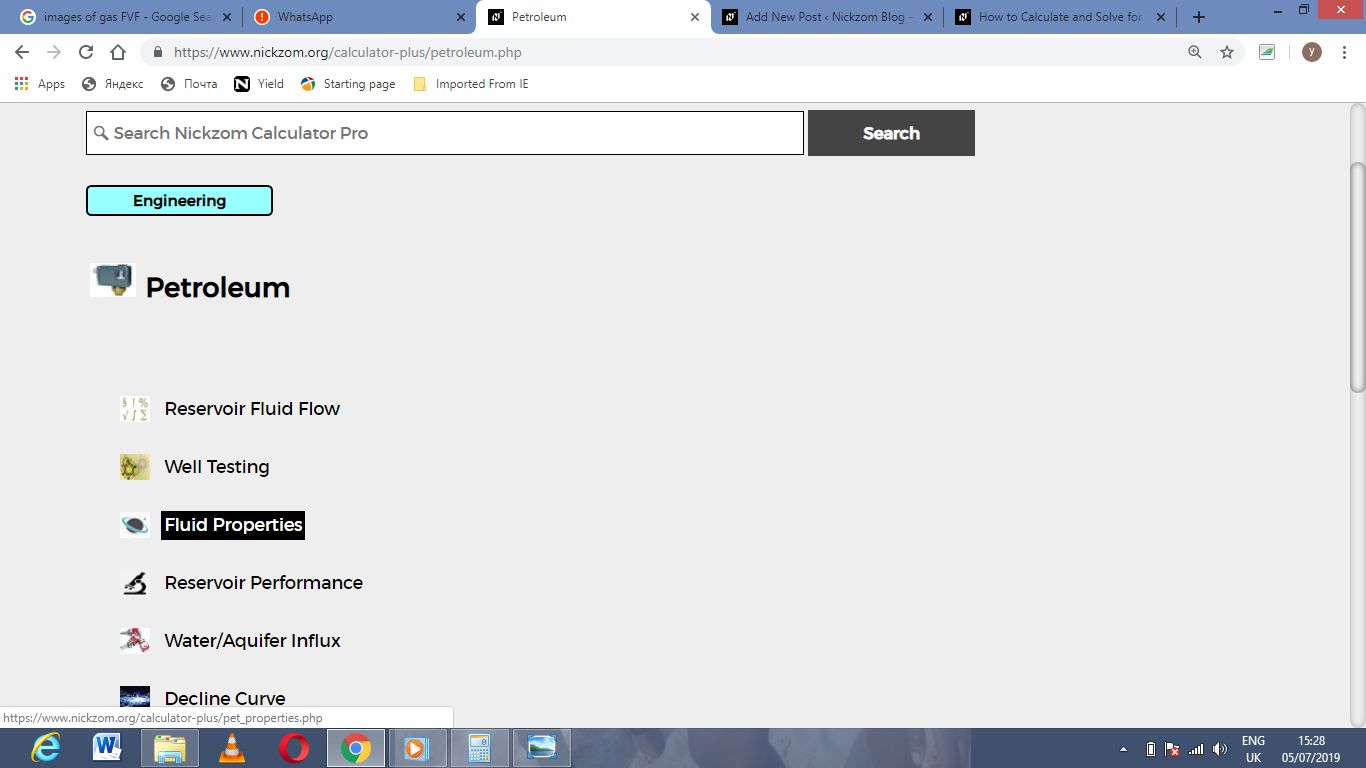Now, Click on Glass Gas Solubility parameter under Fluid Properties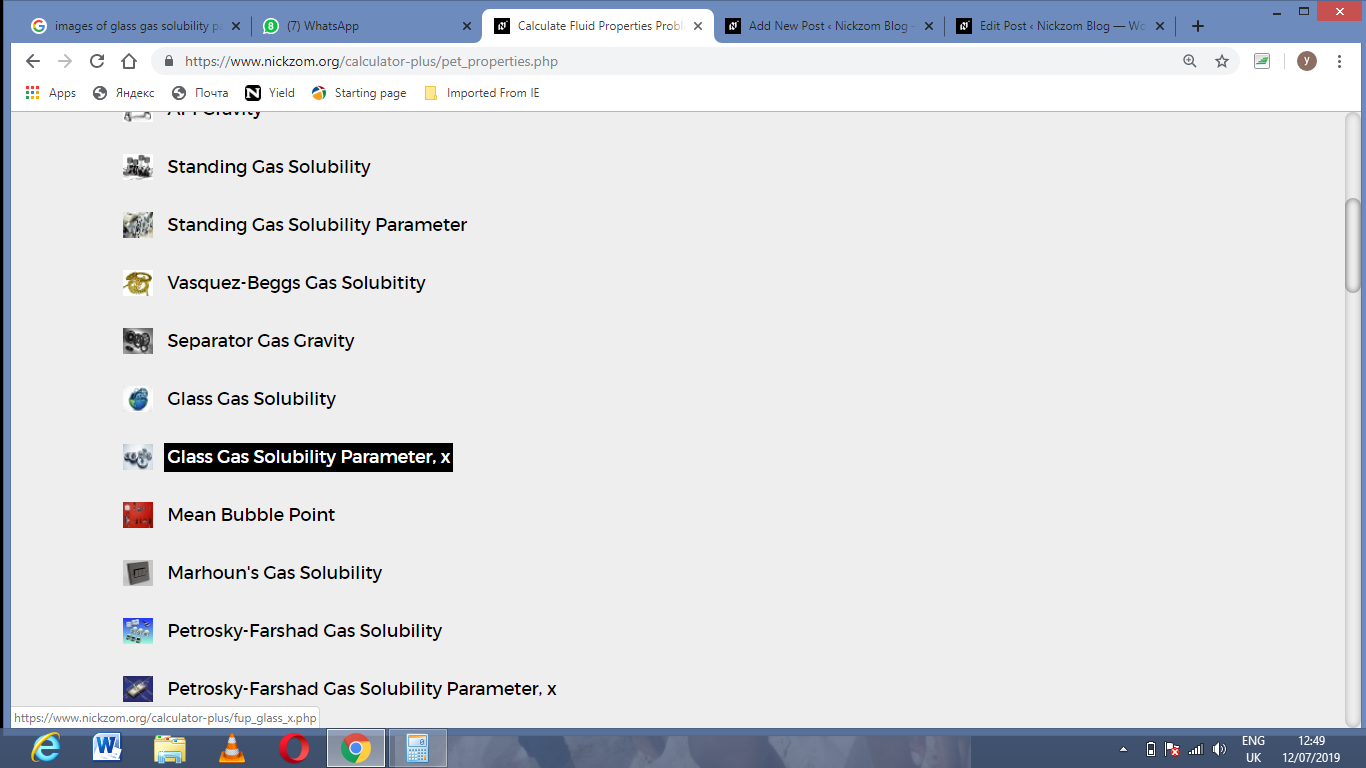The screenshot below displays the page or activity to enter your value, to get the answer for the glass gas solubility parameter according to the respective parameter which are the Pressure (P).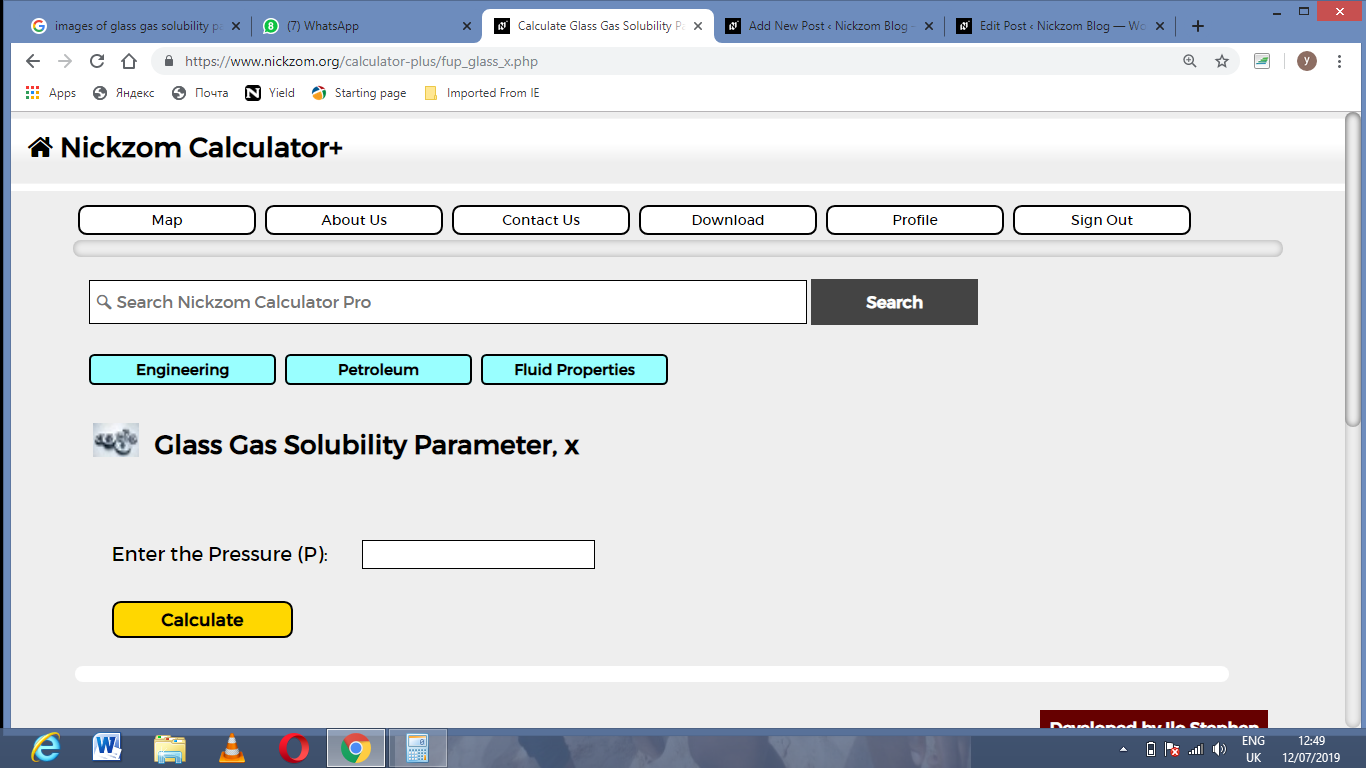Now, enter the value appropriately and accordingly for the parameter is required by the Pressure (P) is 62.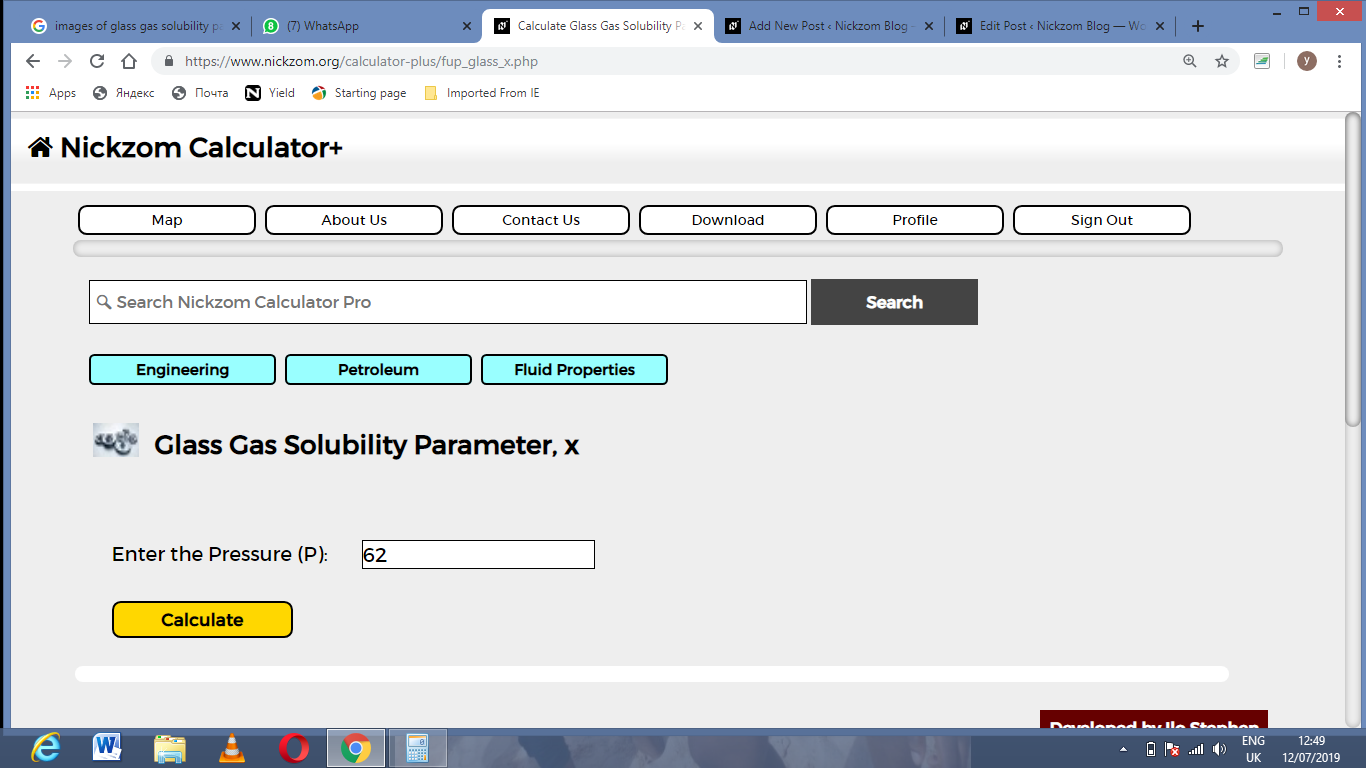Finally, Click on Calculate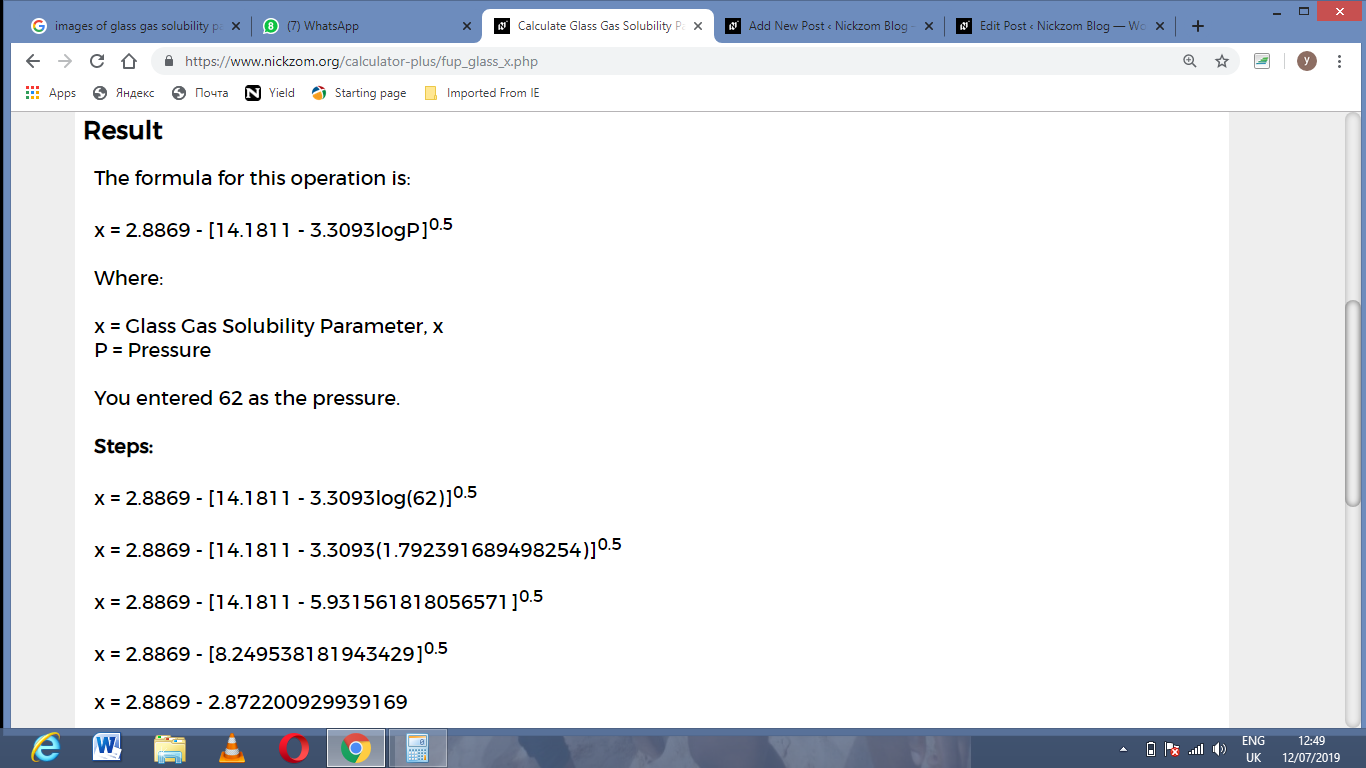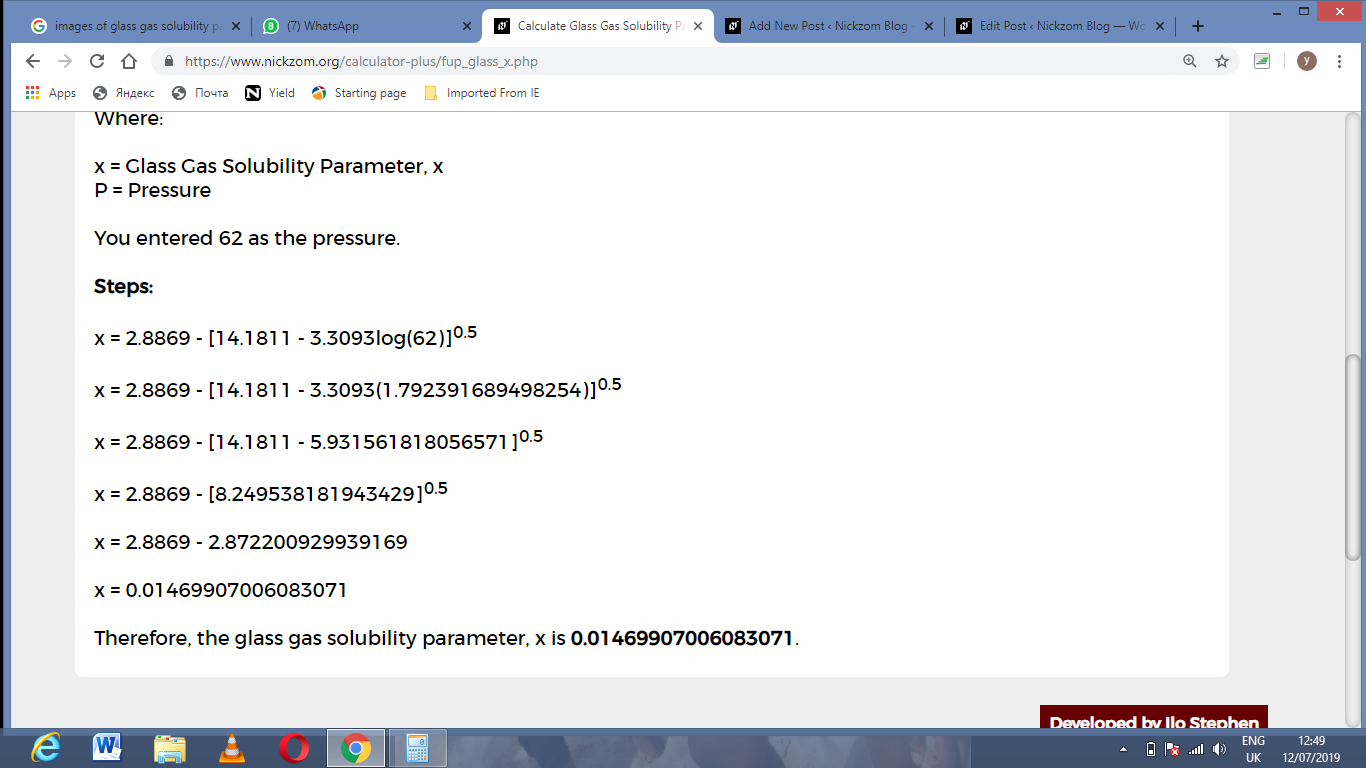As you can see from the screenshot above, Nickzom Calculator– The Calculator Encyclopedia solves for the glass gas solubility parameter and presents the formula, workings and steps too.